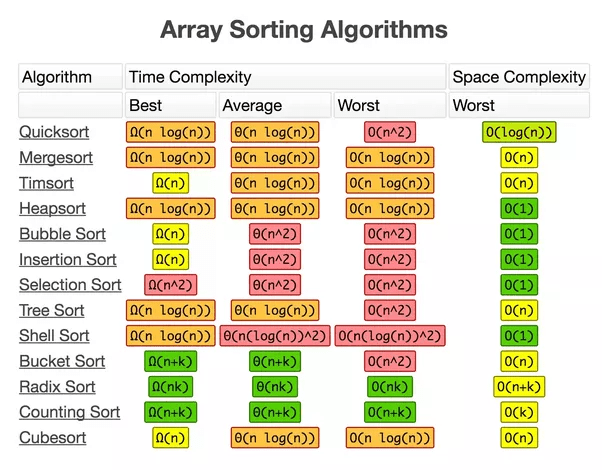In this article, I will try to explain why it’s really important to learn the basics algorithms. The examples will be based on the sorting algorithms.

I suppose that everybody heard about the definition “Big-O”. If you an engineer and you still didn’t hear about it – do not hesitate to google for it. You will understand the importance of it.Many people have heard about such sorting algorithms as Tree Sort, Bubble Sort. But, are they the best? Are they the most optimised? Let’s have a look at the several examples of the sorting algorithms written in Java. In this battle will participate:

1. Bubble Sort – O(n^2)
2. Mergesort – O(n log(n))
3. Counting Sort – O(n+k)
4. Default Java Collections sort method (sorting of primitive int array) – based on Mergesort algorithms regarding the Java doc
5. Default Java Collections sort method (sorting of Integer ArrayList) – based on Mergesort algorithms regarding the Java doc

The input parameter was an array of integer numbers in a range between 0 and 1000. The length of this array is 10 000 000 items.

Below is an example of the unit-test body that was used for measuring the time of CountingSort algorithm. Absolutely the same body was for all the types of algorithms. Of course with the usage of the different algorithms inside 🙂

```int size = 10000000;
int randomIntRange = 1000;
System.out.println("Counting Sorting. Size of array: " + size);

CountingSort sortAlgorithms = new CountingSort();
//MergeSorting sortAlgorithms = new MergeSorting();
//BubbleSorting sortAlgorithms = new BubbleSorting();

//Collecting the integer array
int[] arr = new int[size];
for (int i = 0; i < size; i++) {
arr[i] = new Random().nextInt(randomIntRange);
}

//Measuring the time
long startTime = System.currentTimeMillis();
sortAlgorithms.sort(arr);
long endTime = System.currentTimeMillis();
System.out.println("Time execution: " + (endTime - startTime) + " ms");

```

The results were the next:

1. Mergesort. Size of array: 10000000
Time execution: 1261 ms
2. Counting Sorting. Size of array: 10000000
Time execution: 94 ms
3. Bubble Sorting. Size of array: 10000000
Time execution: endless. Ater waiting of 15 minutes I forgot what do I wait for.

So, as you can see the results are very different.

But then I decided to try to measure a Java implementation of sorting. In the Java doc I found that it is based on Mergesort.
In the 1st test, we collected 10 000 000 integer items into the primitive array of integer, then convert it to the ArrayList and then measure the sorting.
In the 2nd test, we collected 10 000 000 integer items into ArrayList directly.
In both cases we used the Java Collections comparator.

The results were next:

1. Array Java Collections Sorting. Size of array: 10000000
Time execution: 3973 ms
2. Java Collections Sorting. Size of array: 10000000
Time execution: 1715 ms

As you can see the conversion of the array into List also took the time.
But, even so, Counting Sort algorithm is  1715 / 94 ~ 18 times faster.

Now the question – does it make sense to think about the algorithms? The question is open for everybody.

Leave the comments and the feedback!

Happy coding!